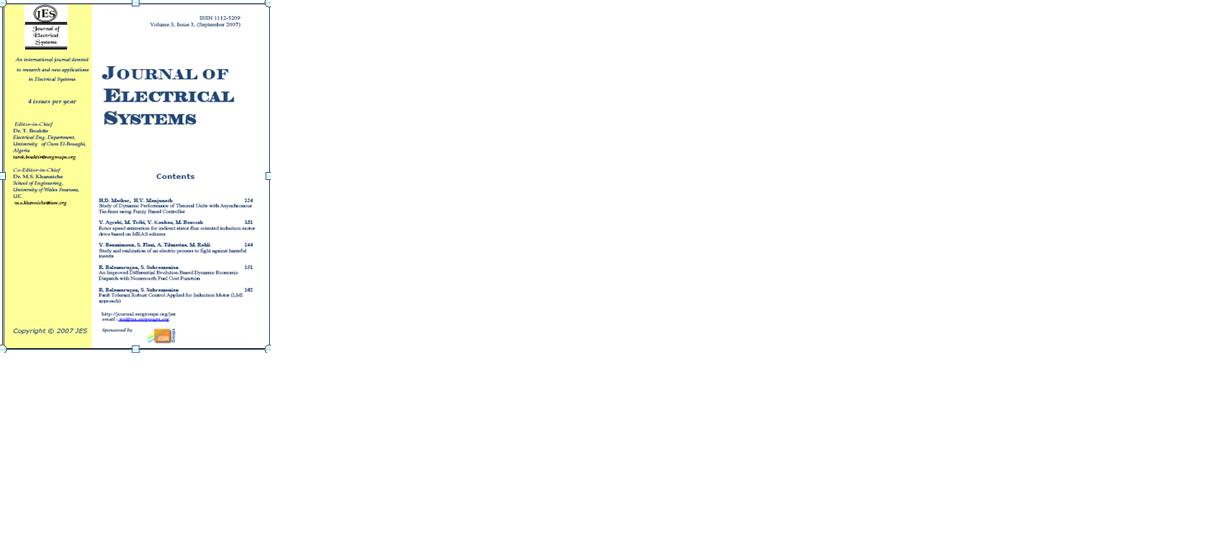##### Accueil>Science et Technologie>Journal of Electrical Systems>Volume 01>Numéro 01> Numéro 01# Numéro 01

## Articles de cette rubrique

### On the Application of TLS Techniques to AC Electrical Drives

This paper deals with the application of a new neuron, the TLS EXIN neuron, to AC induction motor drives. In particular, it addresses two important subjects of AC induction motor drives : the on-line estimation of the electrical parameters of the machine and the speed estimation in sensorless drives. On this basis, this work summarizes the parameter estimation and sensorless techniques already developed by the authors over these last few years, all based on the TLS EXIN. With regard to sensorless, two techniques are proposed : one based on the MRAS and the other based on the full-order Luenberger observer. The work show some of the most significant results obtained by the authors in these fields and stresses the important potentiality of this new neural technique in AC induction machine drives.

### A Simple Fuzzy Logic Approach for Induction Motors Stator Condition Monitoring

Many researches dealt with the problem of induction motors fault detection and diagnosis. The major difficulty is the lack of an accurate model that describes a fault motor. Moreover, experienced engineers are often required to interpret measurement data that are frequently inconclusive. A fuzzy logic approach may help to diagnose induction motor faults. In fact, fuzzy logic is reminiscent of human thinking processes and natural language enabling decisions to be made based on vague information. Therefore, this paper applies fuzzy logic to induction motors fault detection and diagnosis. The motor condition is described
using linguistic variables. Fuzzy subsets and the corresponding membership functions describe stator current amplitudes. A knowledge base, comprising rule and data bases, is built to support the fuzzy inference. The induction motor condition is diagnosed using a compositional rule of fuzzy inference.

### A Practical Fast Acting Control Scheme for Fuzzy Logic-Based Voltage Stabilization Control

This paper presents a simplified control model for stabilizing a load voltage using a switched reactor in parallel with a fixed capacitor of static VAR compensator. Two IGBT’s are used to control the reactance of the switched reactor. A uniform pulse width modulation is used for controlling the two switches. The compensator has a simple control circuit and structure. A complete modeling and numerical simulation for the proposed systems is presented. A high speed Digital Signal Processor is used for implementing proportional-integral (PI) and fuzzy load voltage controllers. Experimental results indicate the superiority of fuzzy logic control over the conventional proportional-integral control method. Simulation results are reported and proved to be in good agreement with the relevant experimental results.

### Solving Harmonics Elimination Problem in Voltage-controlled Three Phase Inverter using Artificial Neural Networks

A novel concept of application of Artificial Neural Networks ( ANN) for generating the optimum switching functions for the voltage and harmonic control of DC-to-AC bridge inverters is presented. In many research, the neural network is trained off line using the desired switching angles given by the classic harmonic elimination strategy to any value of the modulation index. This limits the
utilisability and the precision in other modulation index values. In order to avoid this problem, a new training algorithm is developed without using the desired switching angles but it uses the desired solution of the elimination harmonic equation, i.e. first harmonics are equal to zero. Theoretical analysis of the proposed solving algorithm with neural networks is provided, and simulation results are given to show the high performance and technical advantages of the developed modulator.

### Fuzzy Control of a Lead Acid Battery charger

In this paper, an alternative battery charging control technique based on fuzzy logic for photovoltaic (PV) applications is presented. A PV module is connected to a buck type DC/DC power converter and a microcontroller based unit is used to control the lead acid battery charging voltage. The fuzzy control is used due to the simplicity of implementation, robustness and independence from the complex mathematical representation of the battery. The usefulness of this control method is confirmed by experiments.

| info visites 9078210